output.to from Sideway
Draft for Information Only

# Content

``` Frictional Forces  Dry Friction  Coefficients of Friction  Frictional Force  Angle of Friction  Friction Force and Force Couple```

# Frictional Forces

In general, the applied distributed forces can be in any angle relative to the applied surface at the point of contact. Frictional force is one of the common contact forces found in practical engineering problems. A friction force is a tangential force exected by a surface on the other to prevent the relative lateral motion. Therefore when two surfaces are in contact and the two surfaces move with respect to the other, tangential friction forces are alway developed to resist the motions of two surfaces.

In general, the friction forces can be divided into dry friction and fluid friction. Dry friction or the Coulomb friction is the relative lateral resistance force develope between two solid surfaces.  Fluid friction is the retardation force developed between the layers of fluid causing layers of fluid moving at different velocities.

In practical engineering problems, the forces of action and reaction between two contacting solid surfaces should have components in tangential and normal directions with respect to the contacting surfaces because surfaces in contact are not frictionless. And the dry friction force is the tangential force always acts opposite to the direction of motion of a given body.

## Dry Friction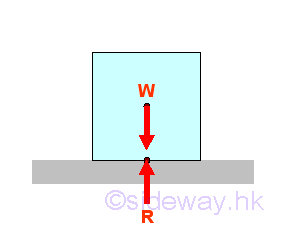Dry friction is the tangential reaction force developed to opposite the applied force of laterial motion. When a solid body of weight W is placed on a plate, there is only a vertical weight component W acting on the plate, and the horizontal component of the body weight is equal to zero. Therefore the reaction of the plate surface also have only the vertical component R and the horizontal component of the reaction is equal to zero.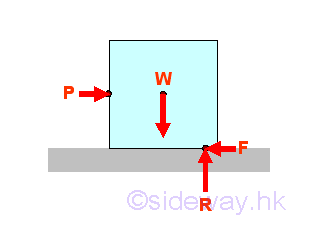When a horizontal force P is applied to the solid body, the solid body will move according to the Newton's second law. But if the applied force P is small, the solid body will not move. And therefore there is always a resultant force to balance the horizontal applied force P. The force to balance the horizontal applied force is the static-friction force F. The solid body can be moved by the applied force P only when the applied force P can not be balanced by the dry friction force.

The nature of the dry friction force is not well understood, but the dry friction foce are usually assumed to be mainly due to the roughness of the contact surfaces, because the irregularities of the contact surfaces cause an additional force to raise the normal weight of the solid body pressing the surfaces together. And therefore the dry friction force is a function of the contact surfaces and the normal component of the reaction of the surfaces.

## Coefficients of FrictionThe dry friction force can be grouped as static friction with no relative motion between the contact surfaces and kinetic friction with relative motion between the contact surfaces.

By experiment, if there is no relative motion between the contact surfaces, a maximum value of static friction force Fs,max for a given pair of contact surfaces is found when the surface of solid body is just about to move. And the maximum static friction force Fs,max is proportional to the normal reaction acting on the contact surfaces. Imply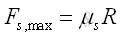where μs  is called the coefficient of static friction and is a constant for a given pair of contact surfaces.

As the solid body start to move, the two contact surfaces move relative to each other also. The magnitude of the dry friction force will drops a little bit because the contact area in contact between the two irregular contact surfaces will be reduced due to the sliding motion. This dry friction force is called kinetic friction force Fk because the dry friction force is the resistive force developed during the relative motion of the two contact surfaces. The kinetic friction force Fk is approximately a constant as the horizontal force increase. Similarly the kinetic friction force Fk is proportional to the normal reaction acting on the contact surfaces also. Imply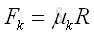where μk  is called the coefficient of kinetic friction and is a constant for a given pair of contact surfaces.

Both the coefficient of static friction and the coefficient of kinetic friction depend on the nature of the contact surface and are highly affected by the condition of the surfaces in contact. Therefore the coefficient of static friction and the coefficient of kinetic friction are usually approximate values. Besides the coefficient of static friction and the coefficient of kinetic friction are dimensionless quantities and do not depend on the area of the surfaces in contact.

For example the coefficients of static friction for the dry surfaces of metal on metal is approximately 0.15 to 0.6 and  the coefficients of static friction for the dry surfaces of metal on wood is approximately 0.2 to 0.6 also because the coefficients of friction are highly affected by the condition of the surfaces in contact. Besides the coefficients of kinetic friction can also be approximated by assuming 25% smaller than the coefficients of static friction.

## Frictional ForceWhen there is no force or the force to move the solid body along the the contact surfaces is equal to zero, there is no dry friction force because the dry friction force is a reaction resistance force to the motion of the solid body along the contact surfaces.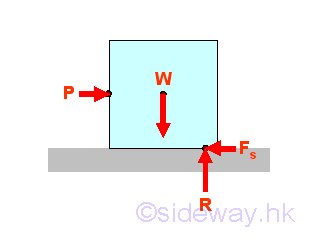When the applied force P used to move the solid body along the contact surfaces is less than the maximum static friction force Fs,max , the static friction force Fs is alway equal in magnitude and opposite in sense to balance the force moving the solid body along the contact surfaces.  implyWhen the motion of solid body is impending.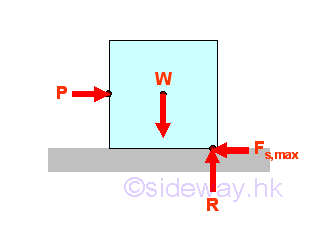When the applied force P used to move the solid body along the contact surfaces is increased to cause the solid body to just about to move, the applied force P is equal to the maximum static friction force Fs,max since the static friction force Fs,max is alway equal in magnitude and opposite in sense to balance the force moving the solid body along the contact surfaces.  imply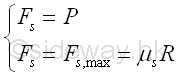When the solid body is sliding.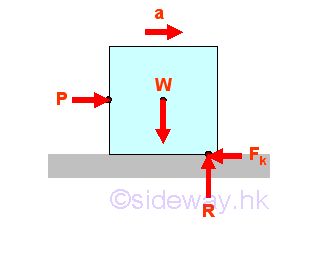When the solid body starts to move along the contact surfaces under the applied force P, the applid force P cannot be balanced by the dry friction force and therefore the equations of equilibrium cannot be applied. However, the dry friction force is still present in between the surfaces in contact as a resistive friction force. The magnitude of the resistive frictional force is now equal to the kinetic friction force Fk which is less than the applied force, but the kinetic friction force is still opposite in sense to the motion of the solid body. imply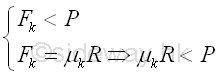Graphically.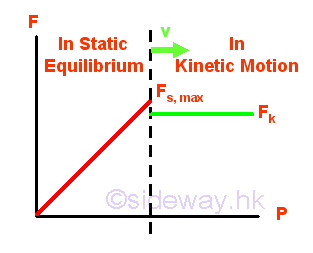## Angle of Friction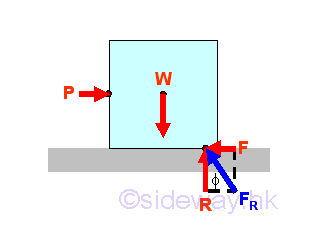Since the dry friction force is a function of normal reaction of the surfaces in contact, the coefficient of friction can be expressed in terms of the angle of the resultant force acting on the surface. Imply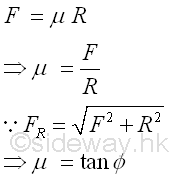Similarly the angle of friction of a solid body isWhen there is no force or the force to move the solid body along the the contact surfaces is equal to zero, there is no dry friction force and the angle of friction is also equal to zero.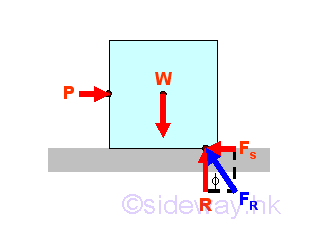When the applied force P used to move the solid body along the contact surfaces is less than the maximum static friction force Fs,max , the static friction force Fs is alway equal in magnitude and opposite in sense to balance the force moving the solid body along the contact surfaces. The angle of friction depends on the magnitude of the horizontal component of the applied force and is equal to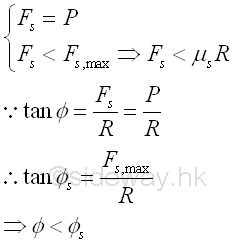When the motion of solid body is impending.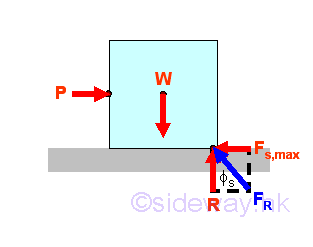When the applied force P used to move the solid body along the contact surfaces is increased to cause the solid body to just about to move, the applied force P is equal to the maximum static friction force Fs,max since the static friction force Fs,max is alway equal in magnitude and opposite in sense to balance the force moving the solid body along the contact surfaces. The value of this angle of friction is also the maximum at static state and is therefore called the angle of static friction. Imply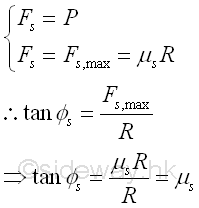When the solid body is sliding.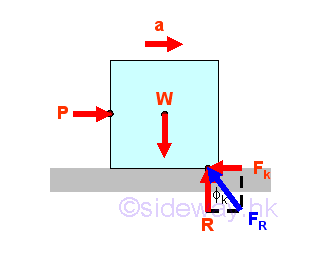When further increasing the applied force P, the solid body starts to move along the contact surfaces under the applied force P, the applid force P cannot be balanced by the dry friction force and therefore the equations of equilibrium cannot be applied. However, the dry friction force is still present in between the surfaces in contact as a resistive friction force. The magnitude of the resistive frictional force is now equal to the kinetic friction force Fk which is less than the applied force, but the kinetic friction force is still opposite in sense to the motion of the solid body. imply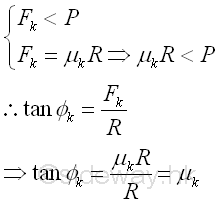## Friction Force and Force CoupleSince the increasing of the applied force from 0 to P will increase the horizontal component of the resultant force FA only which in turn will shift the point of application of the resultant force FA  on the contact surfaces to away from the line of action of the weight W to the corner of block, force couples i.e. couple WR and couple PF are formed. When the block is in static equilibrium, the two force couples are balanced also. Imply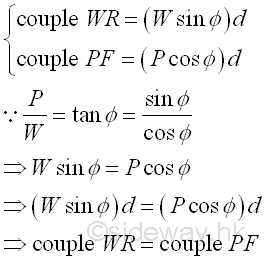Therefore by increasing the magnitude of the applied force P, the maximum possible position of the point of application of the resultant force FA  on the contact surfaces is at the corner of block. ImplyIf the magnitude of the applied force P is less than the maximum value of the static friction force Fs,max when the point of application shifting to the corner of the block, the further increasing the magnitude of the applied force P will tip over the block about the corner of the block because of the formed resultant couple WFR.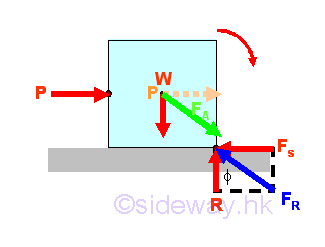ID: 120700007 Last Updated: 7/29/2012 Revision: 0 Ref:References

1. I.C. Jong; B.G. rogers, 1991, Engineering Mechanics: Statics and Dynamics
2. F.P. Beer; E.R. Johnston,Jr.; E.R. Eisenberg, 2004, Vector Mechanics for Engineers: StaticsHome 5

Management

HBR 3

Information

Recreation

Culture

Chinese 1097

English 339

Computer

Hardware 249

Software

Application 213

Latex 52

Manim 205

KB 1

Numeric 19

Programming

Web 289

Unicode 504

HTML 66

CSS 65

SVG 46

ASP.NET 270

OS 429

Python 72

Knowledge

Mathematics

Algebra 84

Geometry 34

Calculus 67

Engineering

Mechanical

Rigid Bodies

Statics 92

Dynamics 37

Control

Natural Sciences

Electric 27